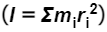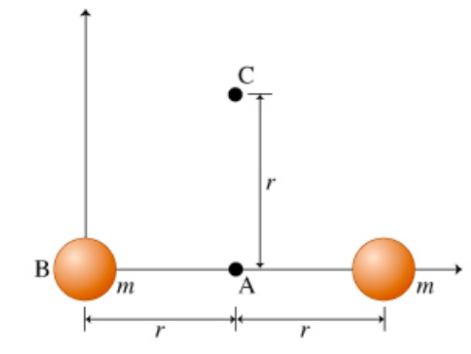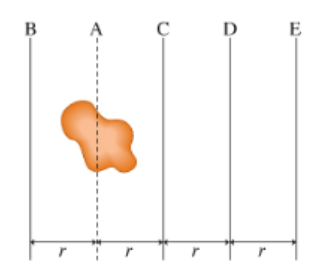###### FREE Expert Solution

This problem applies the parallel axis theorem.

Parallel axis theorem:

$\overline{){\mathbf{I}}{\mathbf{=}}{{\mathbf{I}}}_{\mathbf{c}\mathbf{m}}{\mathbf{+}}{\mathbf{m}}{{\mathbf{d}}}^{{\mathbf{2}}}}$ Where I is the moment of inertia of the object about a parallel axis, Icm is the moment of inertia about the center of mass, and m is the total mass, and d is the distance of the parallel axis.

Moment of inertia of a point mass:

$\overline{){\mathbf{I}}{\mathbf{=}}{{\mathbf{mR}}}^{{\mathbf{2}}}}$ Where m is the mass of the object and r is the distance from the mass to the point of rotation.

X-coordinate of the center of mass:

$\overline{){{\mathbf{x}}}_{\mathbf{c}\mathbf{m}}{\mathbf{=}}\frac{{\mathbf{m}}_{\mathbf{1}}{\mathbf{x}}_{\mathbf{1}}\mathbf{+}{\mathbf{m}}_{\mathbf{2}}{\mathbf{x}}_{\mathbf{2}}\mathbf{+}\mathbf{.}\mathbf{.}\mathbf{.}\mathbf{+}{\mathbf{m}}_{\mathbf{n}}{\mathbf{x}}_{\mathbf{n}}}{{\mathbf{m}}_{\mathbf{1}}\mathbf{+}{\mathbf{m}}_{\mathbf{2}}\mathbf{+}\mathbf{.}\mathbf{.}\mathbf{.}\mathbf{+}{\mathbf{m}}_{\mathbf{n}}}}$ where m1, m2 are masses of particles/objects in the system and x1, x2, are the coordinates of the system.

85% (177 ratings)###### Problem Details

To solve many problems about rotational motion, it is important to know the moment of inertia of each object involved. Calculating the moments of inertia of various objects, even highly symmetrical ones, may be a lengthy and tedious process. While it is important to be able to calculate moments of inertia from the definition, in most cases it is useful simply to recall the moment of inertia of a particular type of object. The moments of inertia of frequently occurring shapes (such as a uniform rod, a uniform or a hollow cylinder, a uniform or a hollow sphere) are well known and readily available from any mechanics text, including your textbook. However, one must take into account that an object has not one but an infinite number of moments of inertia. One of the distinctions between the moment of inertia and mass (the latter being the measure of translational inertia) is that the moment of inertia of a body depends on the axis of rotation. The moments of inertia that you can find in the textbooks are usually calculated with respect to an axis passing through the center of mass of the object. However, in many problems the axis of rotation does not pass through the center of mass. Does that mean that one has to go through the lengthy process of finding the moment of inertia from scratch? It turns out that in many cases, calculating the moment of inertia can be done rather easily if one uses the parallel-axis theorem. Mathematically, it can be expressed as I = Icm + md2, where Icm is the moment of inertia about an axis passing through the center of mass, m is the total mass of the object, and I is the moment of inertia about another axis, parallel to the one for which Icm is calculated and located a distance d from the center of mass. In this problem you will show that the theorem does indeed work for at least one object: a dumbbell of length 2r made of two small spheres of mass m each connected by a light rod (see the figure). NOTE: Unless otherwise noted, all axes considered are perpendicular to the plane of the page.Part A. Using the definition of moment of inertia, calculate Icm, the moment of inertia about the center of mass, for this object. Express your answer in terms of m and r

Icm = ?

Part B. Using the definition of moment of inertia, calculate IB, the moment of inertia about an axis through point B, for this object. Point B coincides with the center of) one of the spheres (see the figure). Express your answer in terms of m and r

IB =
Part C. Now express IB for this object using the parallel-axis theorem. Express your answer in terms of m and r

IB = Icm

Part D. Using the definition of moment of inertia, calculate IC, the moment of inertia about an axis through point C, for this object. Point C is located a distance r from the center of mass (see the figure). Express your answer in terms of m and r

IC=
Part E Now express IC for this object using the parallel-axis theorem. Express your answer in terms of m and r

IC = Icm

Consider an irregular object of mass m. Its moment of inertia measured with respect to axis A (parallel to the plane of the page), which passes through the center of mass (see the second diagram), is given by IA = 0.64mrAxes B, C, D, and E are parallel to axis A; their separations from axis A are shown in the diagram (Figure 2). In the subsequent questions, the subscript indicates the axis with respect to which the moment of inertia is measured: for instance, Ic is the moment of inertia about axis CPart F. Which moment of inertia is the smallest?

a. IA

b. IB

c. IC
d. ID
e. IE

Part G. Which moment of inertia is the largest?

a. IA

b. IB

c. IC
d. ID
e. IE

Part H. Which moments of inertia are equal?
a. IA and ID
b. IB and IC
c. IC and IE
d. No two moments of inertia are equal.

Part I. Which moment of inertial equals 4.64mr2?
a. IB
b. IC
c. ID
d. IE

Part J. Axis X, not shown in the diagram, is parallel to the axes shown. It is known that IX = 6mr2. Which of the following is a possible location for axis X?
a. between axes A and C
b. between axes C and D
c. between axes D and E
e. to the right of axis E